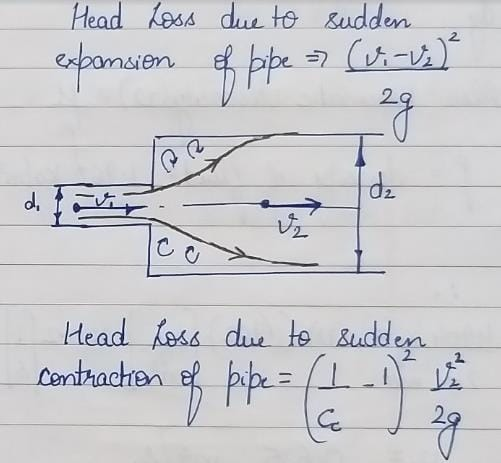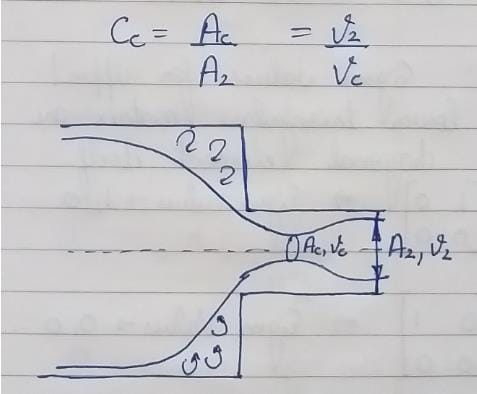Test: Hydraulics & Fluid Mechanics - 1

# Test: Hydraulics & Fluid Mechanics - 1

Test Description

## 25 Questions MCQ Test | Test: Hydraulics & Fluid Mechanics - 1

Test: Hydraulics & Fluid Mechanics - 1 for Civil Engineering (CE) 2023 is part of Civil Engineering (CE) preparation. The Test: Hydraulics & Fluid Mechanics - 1 questions and answers have been prepared according to the Civil Engineering (CE) exam syllabus.The Test: Hydraulics & Fluid Mechanics - 1 MCQs are made for Civil Engineering (CE) 2023 Exam. Find important definitions, questions, notes, meanings, examples, exercises, MCQs and online tests for Test: Hydraulics & Fluid Mechanics - 1 below.
Solutions of Test: Hydraulics & Fluid Mechanics - 1 questions in English are available as part of our course for Civil Engineering (CE) & Test: Hydraulics & Fluid Mechanics - 1 solutions in Hindi for Civil Engineering (CE) course. Download more important topics, notes, lectures and mock test series for Civil Engineering (CE) Exam by signing up for free. Attempt Test: Hydraulics & Fluid Mechanics - 1 | 25 questions in 25 minutes | Mock test for Civil Engineering (CE) preparation | Free important questions MCQ to study for Civil Engineering (CE) Exam | Download free PDF with solutions
 1 Crore+ students have signed up on EduRev. Have you?
Test: Hydraulics & Fluid Mechanics - 1 - Question 1

### One litre of water occupies a volume of

Detailed Solution for Test: Hydraulics & Fluid Mechanics - 1 - Question 1

1000 litre=1 m3=1,000,000 cm3

So 1 litre=1,000,000/1,000=1000cm3.

Test: Hydraulics & Fluid Mechanics - 1 - Question 2

### The value of bulk modulus of a fluid is required to determine

Detailed Solution for Test: Hydraulics & Fluid Mechanics - 1 - Question 2

Alternatively, the Mach Number can be expressed with the density and the bulk modulus for elasticity as,

M = v (ρ/ E)1/2

The bulk modulus elasticity has the dimension pressure and is commonly used to characterize the fluid compressibility.

Test: Hydraulics & Fluid Mechanics - 1 - Question 3

### In a depressed nappe

Detailed Solution for Test: Hydraulics & Fluid Mechanics - 1 - Question 3

This air is carried away by the flowing water, which results in creating a negative pressure beneath the nappe. The negative pressure drags the lower side of the nappe towards the surface of the weir wall. This results in more discharge than the normal discharge. In order the keep the atmospheric pressure in the space below the nappe holes are made through the channel walls which are connected through the pipes to the atmosphere as shown in figure. Such holes are called 'Ventilation' of a weir.

Test: Hydraulics & Fluid Mechanics - 1 - Question 4

In one dimensional flow, the flow

Detailed Solution for Test: Hydraulics & Fluid Mechanics - 1 - Question 4

For one dimensional flow, the velocity is the function of single coordinate (i.e) u= f(x).

Let u be the velocity.

The function u = f(x) is the straight line.

Therefore one dimensional flow is the straight line flow.

Test: Hydraulics & Fluid Mechanics - 1 - Question 5

The kinematic viscosity is the

Test: Hydraulics & Fluid Mechanics - 1 - Question 6

The diameter of the nozzle (d) for maximum transmission of power is given by (where D = Diameter of pipe, f = Darcy 's coefficient of friction for pipe, and l = Length of pipe )

Test: Hydraulics & Fluid Mechanics - 1 - Question 7

The discharge over a right angled notch is (where H = Height of liquid above the apex of notch)

Test: Hydraulics & Fluid Mechanics - 1 - Question 8

A weir is said to be broad crested weir, if the width of the crest of the weir is __________ half the height of water above the weir crest.

Test: Hydraulics & Fluid Mechanics - 1 - Question 9

An error of 1% in measuring head over the apex of the notch (H) will produce an error of __________ in discharge over a triangular notch.

Test: Hydraulics & Fluid Mechanics - 1 - Question 10

The length AB of a pipe ABC in which the liquid is flowing has diameter (d1) and velocity v1 THe pipe is suddenly enlarged to diameter (d2) at B and velocity reduced to v2 which is constant for the length BC. The loss of head due to sudden enlargement is

Detailed Solution for Test: Hydraulics & Fluid Mechanics - 1 - Question 10Test: Hydraulics & Fluid Mechanics - 1 - Question 11

Coefficient of contraction is the ratio of

Test: Hydraulics & Fluid Mechanics - 1 - Question 12

In a free nappe,

Test: Hydraulics & Fluid Mechanics - 1 - Question 13

The Reynold's number of a ship is __________ to its velocity and length.

Test: Hydraulics & Fluid Mechanics - 1 - Question 14

When a tube of smaller diameter is dipped in water, the water rises in the tube due to viscosity of water.

Detailed Solution for Test: Hydraulics & Fluid Mechanics - 1 - Question 14

It is due to the capillary action in which the water rises in the tube due more adhesive force betwwen the tube surface and water tham the water-water cohesive force

Test: Hydraulics & Fluid Mechanics - 1 - Question 15

The discharge over a rectangular notch is (where b = Width of notch, and H = Height of liquid, above the sill of the notch)

Test: Hydraulics & Fluid Mechanics - 1 - Question 16

The discharge through a siphon spillway is

Test: Hydraulics & Fluid Mechanics - 1 - Question 17

The maximum discharge over a broad crested weir is

Detailed Solution for Test: Hydraulics & Fluid Mechanics - 1 - Question 17

Actual Formula for Discharge for broad crested weir is Q = Cd (g)0.5 b2/3 H3/2

For MaximumDischarge = (9.81)1/2 (2/3)3/2 = 1.7048~1.71(approx).

Test: Hydraulics & Fluid Mechanics - 1 - Question 18

In a venturimeter, the velocity of liquid at throat is __________ than at inlet.

Detailed Solution for Test: Hydraulics & Fluid Mechanics - 1 - Question 18

Discharge stands for Q=A*V.
As water enters through inlet, it has larger area in comparison to "throat" of venturimeter. Water entered is assumed to have zero losses in pipe and venturimeter so, discharge at inlet should be equal to discharge outlet. As discharge is same throughout pipe and venturimeter and area of throat is lesser than inlet & outlet both, its velocity will be higher at throat than at inlet & outlet both( Q= const. so Velocity is inversely proportional to Area).

Test: Hydraulics & Fluid Mechanics - 1 - Question 19

The loss of head due to friction in a pipe of uniform diameter in which a viscous laminar flow is taking place, then coefficient of friction is (where RN = Reynold number)

Detailed Solution for Test: Hydraulics & Fluid Mechanics - 1 - Question 19

Coefficient of friction= Friction Factor/4

Darcy Weisbach friction factor for laminar flow = 64/Rn

Coefficient of friction= 16/Rn

Test: Hydraulics & Fluid Mechanics - 1 - Question 20

Which of the following is an example of laminar flow?

Test: Hydraulics & Fluid Mechanics - 1 - Question 21

The pressure less than atmospheric pressure is known as

Test: Hydraulics & Fluid Mechanics - 1 - Question 22

The total energy line lies over the hydraulic gradient line by an amount equal to the

Detailed Solution for Test: Hydraulics & Fluid Mechanics - 1 - Question 22

From equation 1 and 2 it is clear that. TEL will be lead to HGL by velocity head.

Test: Hydraulics & Fluid Mechanics - 1 - Question 23

A structure whose width is __________ the width of the channel, is called a flumed structure.

Test: Hydraulics & Fluid Mechanics - 1 - Question 24

The maximum efficiency of transmission through a pipe is

Detailed Solution for Test: Hydraulics & Fluid Mechanics - 1 - Question 24

The hydraulic power can be transmitted by a pipeline. For a maximum power transmission, the head due to friction in the flow equals to one third of the head at source to be transmitted. The maximum power transmitted efficiency is 67%.

Test: Hydraulics & Fluid Mechanics - 1 - Question 25

The coefficient of viscosity may be determined by

Information about Test: Hydraulics & Fluid Mechanics - 1 Page
In this test you can find the Exam questions for Test: Hydraulics & Fluid Mechanics - 1 solved & explained in the simplest way possible. Besides giving Questions and answers for Test: Hydraulics & Fluid Mechanics - 1, EduRev gives you an ample number of Online tests for practice(Scan QR code)